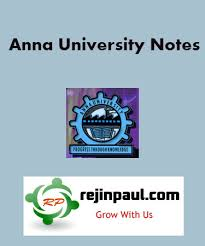## CS8251 Programming in C Syllabus Notes Question Paper Question Banks Anna University - Regulation 2017 2nd Semester Notes CBCS

Anna University Programming in C Syllabus Notes Question Bank Question Papers
Anna University CS8251 Programming in C Notes are provided below. CS8251 Notes all 5 units notes are uploaded here. here CS8251 Programming in C notes download link is provided and students can download the CS8251 Lecture Notes and can make use of it.CS8251 Programming in C Syllabus Regulation 2017
UNIT I BASICS OF C PROGRAMMING
Introduction to programming paradigms - Structure of C program - C programming: Data Types – Storage classes - Constants – Enumeration Constants - Keywords – Operators: Precedence and Associativity - Expressions - Input/Output statements, Assignment statements – Decision making statements - Switch statement - Looping statements – Pre-processor directives - Compilation process
UNIT II ARRAYS AND STRINGS
Introduction to Arrays: Declaration, Initialization – One dimensional array – Example Program: Computing Mean, Median and Mode - Two dimensional arrays – Example Program: Matrix Operations (Addition, Scaling, Determinant and Transpose) - String operations: length, compare, concatenate, copy – Selection sort, linear and binary search
UNIT III FUNCTIONS AND POINTERS
Introduction to functions: Function prototype, function definition, function call, Built-in functions (string functions, math functions) – Recursion – Example Program: Computation of Sine series, Scientific calculator using built-in functions, Binary Search using recursive functions – Pointers – Pointer operators – Pointer arithmetic – Arrays and pointers – Array of pointers – Example Program: Sorting of names – Parameter passing: Pass by value, Pass by reference – Example Program: Swapping of two numbers and changing the value of a variable using pass by reference.
UNIT IV STRUCTURES
Structure - Nested structures – Pointer and Structures – Array of structures – Example Program using structures and pointers – Self referential structures – Dynamic memory allocation - Singly linked list - typedef
UNIT V FILE PROCESSING
Files – Types of file processing: Sequential access, Random access – Sequential access file - Example Program: Finding average of numbers stored in sequential access file - Random access file - Example Program: Transaction processing using random access files – Command line arguments